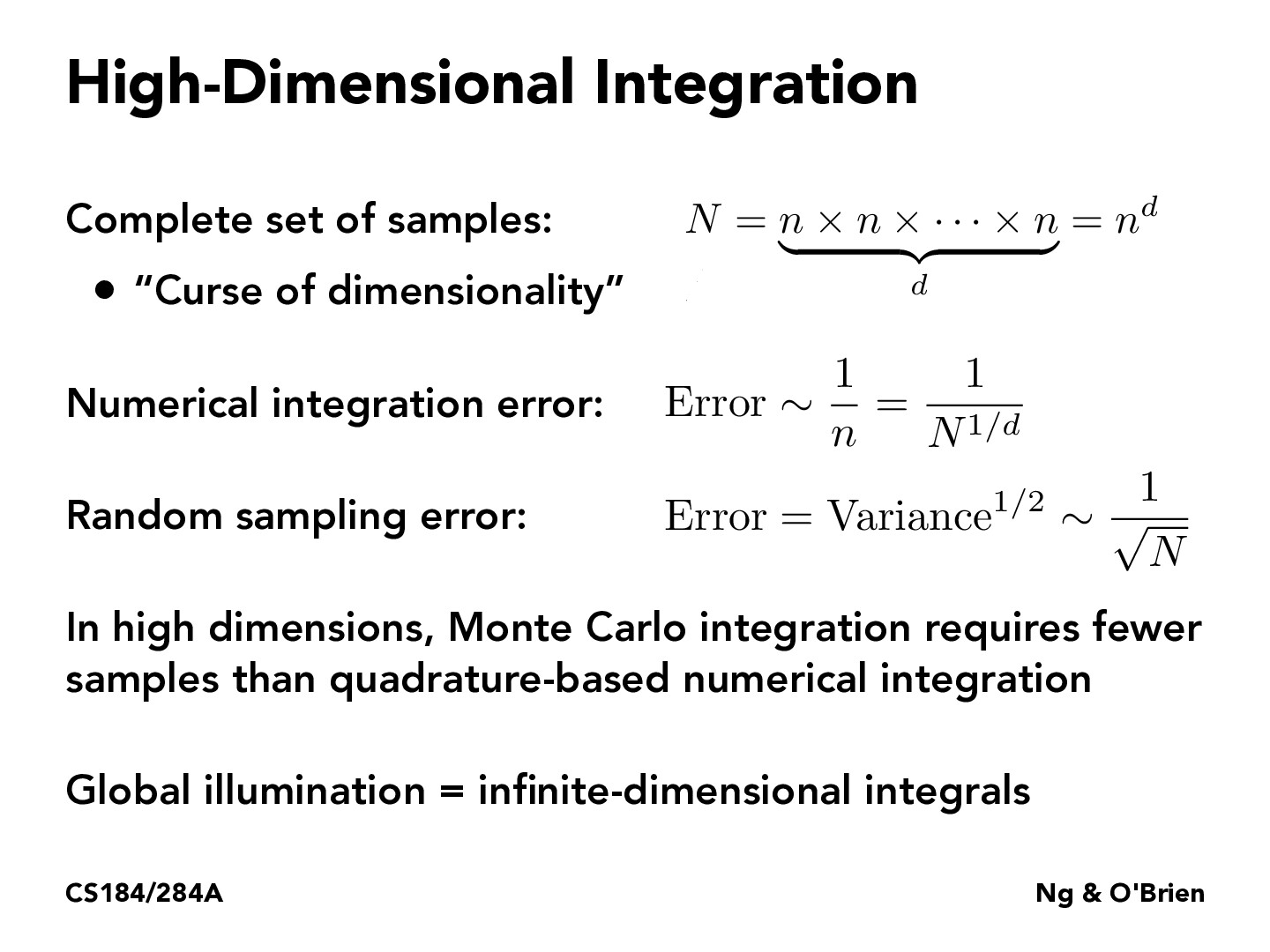Lecture 12: Monte Carlo Integration (9)rishiarjun

Is it fair to say that we would use quadrature-based numerical integration for low dimensions due to its speed and the lack of randomness, and in contrast, we use Monte Carlo integration for only high dimensions? As it says in the slide, it uses fewer samples so does that mean its more efficient at a high dimension?yzliu567

MC integration has a lower variance if we have a closer guess to the integrand compared to an uniform distribution. I think even if we are in low dimension, if the distribution is far from uniform, MC integration is a better idea.ShaamerKumar

@yzliu567 would you be able to explain why MC integration always has a lower variance?ShaamerKumar

@yzliu567 would you be able to explain why MC integration always has a lower variance?yzliu567

@ShaamerKumar Sorry. I shouldn't have used the word 'always' because I couldn't come up with a rigorous mathematical proof, (and this statement depneds on how we measure the 'closeness' between distributions). But this can be explained intuitively. When $p$ is closer to $f$, the random variable $\frac{f(x)}{p(x)}$'s variance should be smaller because the distribution is flatter.rsha256

Why do we divide by $N$ and not $N-1$ in the denominator? Do we not have to worry about it being a biased/unbiased estimate?

You must be enrolled in the course to comment